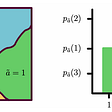# Analysis of “Cancer Diagnosis Dataset”

Basic overview about the domain knowledge with respect to Cancer -

## What cancer is?

Our body is made up of billions of cells,they are continuously dying and regenerating.Normally,a cell divides and make a perfect copy of itself using a genetic blueprint called DNA.Once in a while DNA blueprint gets damaged sometimes so that cell doesn’t listen to body signals and keeps on dividing forming a tumor.

## What is business problem we needed to solve?

Let us discuss briefly about data and business problem.Because it is very important to understand the business problem we are solving.

When a patient seems to have a cancer ,we take a cancer tumor from the patient and we go through genetic sequencing of DNA of tumor.Once sequenced ,a cancer tumor can have thousands of genetic mutations.Here briefly a ‘mutation’ is small change in gene which causes cancer.One more important thing is for every gene there is a variation associated with it.Now with the help of gene and variation we have to classify which class(total we have 9 classes) it belongs to.Only some classes belongs to cancer.

## My goal is to analyze the Cancer Diagnosis data set taken from the “Kaggle”.

Problem / objective : Analyze the data set and predict the probability of each data point belonging to one of nine classes given in the data set.

Following the step by step process to analyze the data set:

1. Overview on Dataset
2. How to convert/map the real world problem to ML problem?
3. Data pre-processing
4. Training and Testing
5. Performance evaluation metrics
6. Exploratory data alaysis
7. Modeling
8. Prediction

## DATA OVERVIEW:

• Source: https://www.kaggle.com/c/msk-redefining-cancer-treatment/data
• We have two data files: one contains the information about the genetic mutations and the other contains the clinical evidence (text) that human experts/pathologists use to classify the genetic mutations.
• First data file training_variant has four columns ID, Gene , Variations, Class
• Second data file training_text contains two columns ID,Text
• Both these data file have same ID

Those of us who does not belong to medicine background it is better to go through the following link that give better understanding of the data

## MAPPING THE REAL WORLD PROBLEM TO ML PROBLEM.

There are nine different classes the genetic mutation can be classified into so,it is multi-class classification problem.

## Data pre-processing:

Some default constraints we should know before processing the data in ML. Since based on this data set and its problem, below are the constraints I’m following:

• Interpretability

The interpretability is very important in this type of problems where the errors are very costly.Let’s say that in the nine classes [0–9] the probability of datapoint belongs to class 1 is 50% and class 2 is %20 and 3 is 30% .Assume if one class has the high probability our model will declare that patient have cancer. In this case our patient have only 50% chance of getting cancer but our model will declare that he has cancer that is a huge error.If our model is interpret-able then the doctor could see the probabilities of each class and he will decide whether the patient have cancer or not.

• Class probabilities
• Penalize the errors
• No Latency

The doctors has to go through lots and lots of research papers to detect whether the patient have cancer or not. so, our model could take some time to give the output. Like 1 min or even 5 min.

Firstly, let’s import the necessary Python libraries. NLTK is pretty much the standard library in Python library for text processing, which has many useful features.

`import pandas as pdimport matplotlib.pyplot as pltimport reimport timeimport warningsimport numpy as npfrom nltk.corpus import stopwordsfrom sklearn.decomposition import TruncatedSVDfrom sklearn.preprocessing import normalizefrom sklearn.feature_extraction.text import CountVectorizerfrom sklearn.manifold import TSNEimport seaborn as snsfrom sklearn.neighbors import KNeighborsClassifierfrom sklearn.metrics import confusion_matrixfrom sklearn.metrics.classification import accuracy_score, log_lossfrom sklearn.feature_extraction.text import TfidfVectorizerfrom sklearn.linear_model import SGDClassifier`

Next, we can import the training_variant file and store it in a Pandas dataframe called data .

Since we have two data files let’s import the training_text file and store it in pandas

Data pre-processing

## preprocessing of text data by using nltk importing stopwords.(stopwords removal)

stopwords remove the unwanted and useless words from the text data example “this pasta is very tasty” stopwords are ‘this’ ‘is’ ‘very’ ,and it Reduces the data a lot and reduces the complexity of the problem as well.

Since the id of the two data files is same let’s merge the two data file into one data file using simple merge operation(.merge())

Next we will convert the text data into numerical form.

VECTORIZATION : conversation of text data into vector form is called vectorization.

At the moment, we have our text as lists of tokens (also known as lemmas). To enable Scikit-learn algorithms to work on our text, we need to convert text into a vector.For this we can use one one-hot encoding or response encoding or mean value replacement.we have features like gene,variation,text.

In this we use response coding,it converts each of the gene into a vector with class labels where each of the values are probability values with some Laplace-smoothing. For some models response coding works better and for some models one hot encoding works better

with this the entire gene feature is converted into numerical vector,do same thing on variation and text convert them into numerical vectors.

## Train Test and Cross Validation split

In this section we will break down our dataset into 3 parts(train, test, cross validation).One is for training our model.second is for testing the model and next one is kept hidden to our model during training.Now we will use the test part for testing and we will find the accuracy of the model.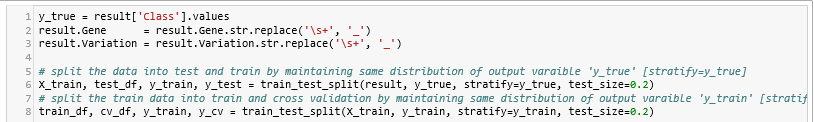We split the data into train, test and cross validation data sets, preserving the ratio of class distribution in the original data set

# Prediction

## Performance Metrics

Metrics:

• Multi class log-loss
• Confusion matrix

Data Visualization :

## EXPLORATORY DATA ANALYSIS

It (EDA) is an approach to analyzing data sets to summarize their main characteristics, often with visual methods. A statistical model can be used or not, but primarily EDA is for seeing what the data can tell us beyond the formal modeling or hypothesis testing task.

Splitting the data:-As the data is not temporal in nature which means not changing with time we can split the data randomly for training ,cross validation and testing.Then after splitting the data it is also found out that training and test data having almost same distributions and from distributions it is clear that data is imbalanced.

As we know that log-loss is ranging from 0 to infinite.So we first defined one random model so that if our ML model has log-loss less than our random model then we can consider our ML model is good.After giving data to our random model it is given log loss roughly 2.5.Even we checked precision and recall matrix in which diagonal elements(which are precision and recall of all classes) which seemed to be very low because of a random model.Precision and recall matrices are attached below.From the above distributions it is also very clear that 1,2,4,7 classes are majority classes.

# using a ‘Random’ Model

In a ‘Random’ Model, we generate the NINE class probabilities randomly such that they sum to 1.It is better to use a small trick like this apply random classifiers and perform few metrics like precision and recall etc that gives better intuition of the building a model.

Now we have seen working of random model by this we understood that diagonal elements for log loss should be maximum.Perform uni-variate analysis on all the features and data points as well .

UNIVARIATE ANALYSIS:

We took each feature and checked whether it is useful for predicting in class label by various ways so that we can use that feature if it is not we can simply remove that feature

1. Gene Feature

As we know that gene is categorical feature from that we observed that there are 235 types of unique genes out of which top 50 genes nearly contribute 75 percent of data.

Now we featured the gene into vector by one hot encoding and response coding.Then we built one simple model Logistic Regression with Calibrated Classifier and applied gene feature and class labels to it.We found out that Train,CV,Test log-loss values are roughly same and also found out that log loss value is less than 2.5(Random Classifier value) .Hence we can say that Gene is an important feature for our classification.We can also conclude that gene is stable feature because CV and Test errors are roughly equal to Train Errors

2.Variation Feature

Here variation is also a categorical feature and we observed that 1927 unique variations out of 2124 present in training data which means most of variations occurred once or twice. CDF of variations looks as follows:

Cumulative Distribution is straight line which means most variations occur once or twice in training data.We featured the Variation into vector by one hot encoding and response coding.As we did earlier for gene feature we built a simple model LR and applied data to it and found that log loss values of Train,CV,Test found to be less than Random Model.But the difference between Train log loss and CV,Test log loss is significantly more than gene feature which means variation feature is unstable.But as the log loss is less than the Random Model we still use the variation feature but be careful it is not stable.

3.Text Feature

In text data there are total 53,000 unique words present in training data.And also observed that most words occur very few times which is common in text data.We converted the text data into vector by BOW(one hot encoding) and Response Coding.As we did in previous cases we applied it to simple model LR and found out log loss values of Train,CV,Test found to be less than Random Model.From the distributions of CV,Test data it is found out that test feature is a stable feature.

Now combine all the features by two ways

1. One hot encoding: It is found out that by one hot encoding the dimensionality is 55,517 which is because of text data.
2. Response Coding: It is found out that by Response Coding the dimensionality became 27 (each feature corresponds to 9 dimensions).

other MODELS : In this case i am using K-NN ,test on multiple model and then you will come to know which model is performing well. find more about KNeighborsClassifier() here http://scikit-learn.org/stable/modules/generated/sklearn.neighbors.KNeighborsClassifier.html

Lets say k=5 in the above figure it calculates five the nearest neighbors by measuring the distances and take the majority vote if there are 4 red and one blue(lets say).It will declare the box as red.

In confusion matrix u can see that diagonal elements are maximum so as we know the class 1,2,4,7 has high distribution,here in confusion matrix it shows clearly that those 1,2,4,7 has high distribution.In the above the log loss is 1.130 and the mis-classified points are 0.394.U can observe clearly that our k-NN is better than random model.

Sample query point to K-NN

In the above output our k-NN model does well, you can see that our actual class and the predicted class are same.U can see that frequency of points for class 7 is 10 we have taken the tuning parameter k=15,since k-NN work on majority vote it give class seven as output.But k-NN is not recommended because it has less interpret-able.

Sample query 2

In the above u can see that our predicted class is 7 but actual class is 4 and all the other classes have only one nearest neighbor.If this was given to the doctor,think that actual class is 7 because majority of neighbors belongs to class 7. Because he can only see the nearest neighbors nothing other than that k-NN does not have feature importance it is less interpret able.Hence k-NN is does not perform well for this kind of applications.

# Using Logistic regression with class balancing

Logistic regression is one of classification techniques that is used used extensively in handling categorical data.

In logistic regression we prefer to do one hot encoding since logistic regression works very well for high dimensional data.We use sgdclassifier as long as our metric is log loss it acts as logistic regression and our hyper parameter is alpha( lambda in logistic regression).when we do class balancing what we do is oversampling minority classes will get respect even that is the beauty of logistic regression with class balancing.

In the above u can see that mis-classified points are significant less than k-NN,and log loss is similar to k-NN.

lg is highly interpret-able because u can use the weights for feature importance.Because u r doing class balancing some of the minority classes like 8,9 values also perform very well.

In the above u can see that our actual and predicted classes are same.

let’s see the incorrectly classified points

u can see in the code snippet that our model incorrectly predicted the class, we can easily get fooled in some of these cases.Remember our models are not prefect, u can get that by looking at precision and recall.In precision and recall u can see 68% of the times we are getting correct results by using logistic regression .

From the above we can see that logistic regression is better than k-NN.

CONCLUSION.

“This is one of the hardest problem, if this is the easy problem we would have solved some of the complex medical problems in the history of mankind. If so first we would prefer Cancer.Machine learning is just helping cancer diagnosis, it’s not solving the whole problem, solving the whole problem effectively means all the values in should be one.if can,it could make humongous impact on humanity.we are not yet there, but we are making some small progress in that direction but it’s still a small progress.Even getting the log loss with this low is a miracle.Imagine if 68% of the times i am correctly classifying that means a lot for the pathologist because today the pathologist’s are spending hundred hours to evaluate the gene and variations 68 hours in that effort can be automated now.”

--

--

--

Engineer, problem solver, tech enthusiast

Love podcasts or audiobooks? Learn on the go with our new app.

## Part II: What is Attention?## Introduction to Multimodal Deep Learning## Master Machine Learning And NLP Through SpaceX’s Dragon Launch And… Twitter?## Similar Texts Search In Python With A Few Lines Of Code: An NLP Project## What it takes to deploy a model## Building Spam Message Detector Using Python and Vue JS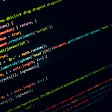## Representation Learning: A Key Idea of Deep Learning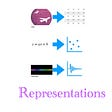Engineer, problem solver, tech enthusiast

## Girvan Newman Part 3 — Two Multi-Edge Removal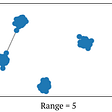## Random Variable — Probability for Data Science